Function Repository Resource:

# FractionalD

Calculate a fractional derivative

Contributed by: Sami Yrjänheikki
 ResourceFunction["FractionalD"][f,{x,α}] gives the fractional derivative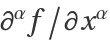.

## Details and Options

If α is an integer, ResourceFunction["FractionalD"][f,{x,α}] is equivalent to D[f,{x,α}].
The Cauchy method uses the formula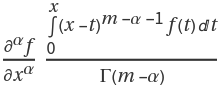.
The Caputo method uses the formula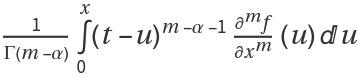.

## Examples

### Basic Examples (2)

Half-derivative of x2:

 In:=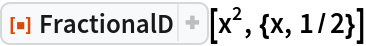Out=Taking the half-derivative twice yields the usual first derivative:

 In:=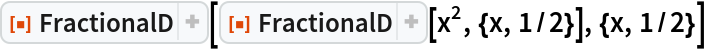Out=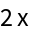### Scope (5)

If α is an integer, the result is the normal derivative:

 In:=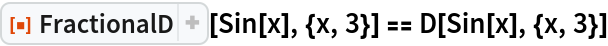Out=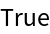Half-derivative of elementary functions:

 In:=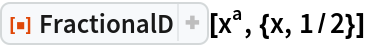Out=In:=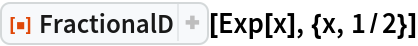Out=In:=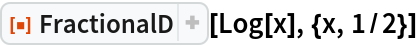Out=Half-derivative of trig functions:

 In:=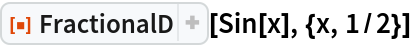Out=In:=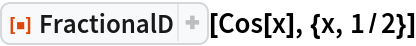Out=Half-derivative of inverse trig functions:

 In:=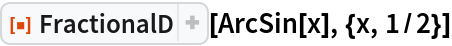Out=In:=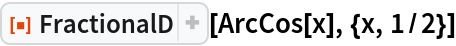Out=A more exotic fractional derivative:

 In:=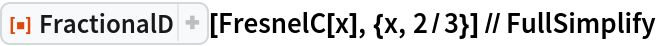Out=### Options (1)

#### Method (1)

Choosing between Cauchy and Caputo may result in performance differences:

 In:=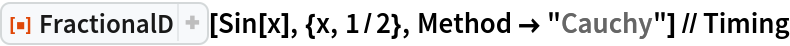Out=In:=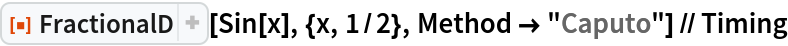Out=### Possible Issues (1)

FractionalD uses integration under the hood. Sometimes the integral cannot be computed:

 In:=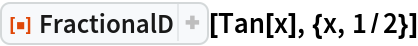Out=### Neat Examples (1)

The fractional derivative smoothly interpolates between the function and its derivative:

 In:=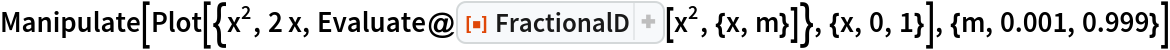Out=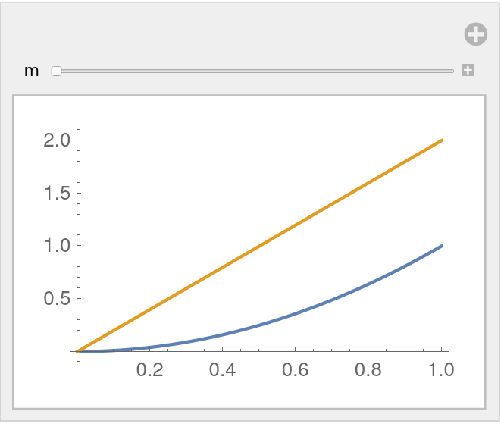Sami Yrjänheikki

## Version History

• 1.0.0 – 15 April 2020